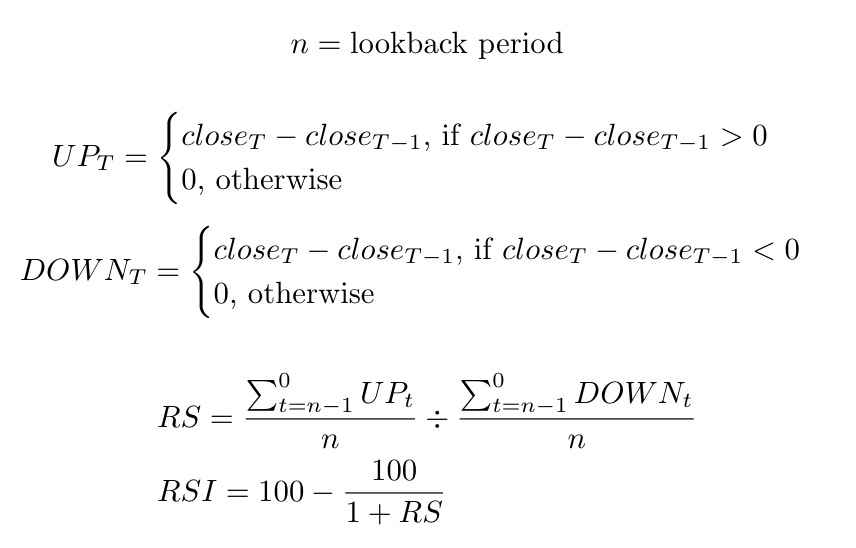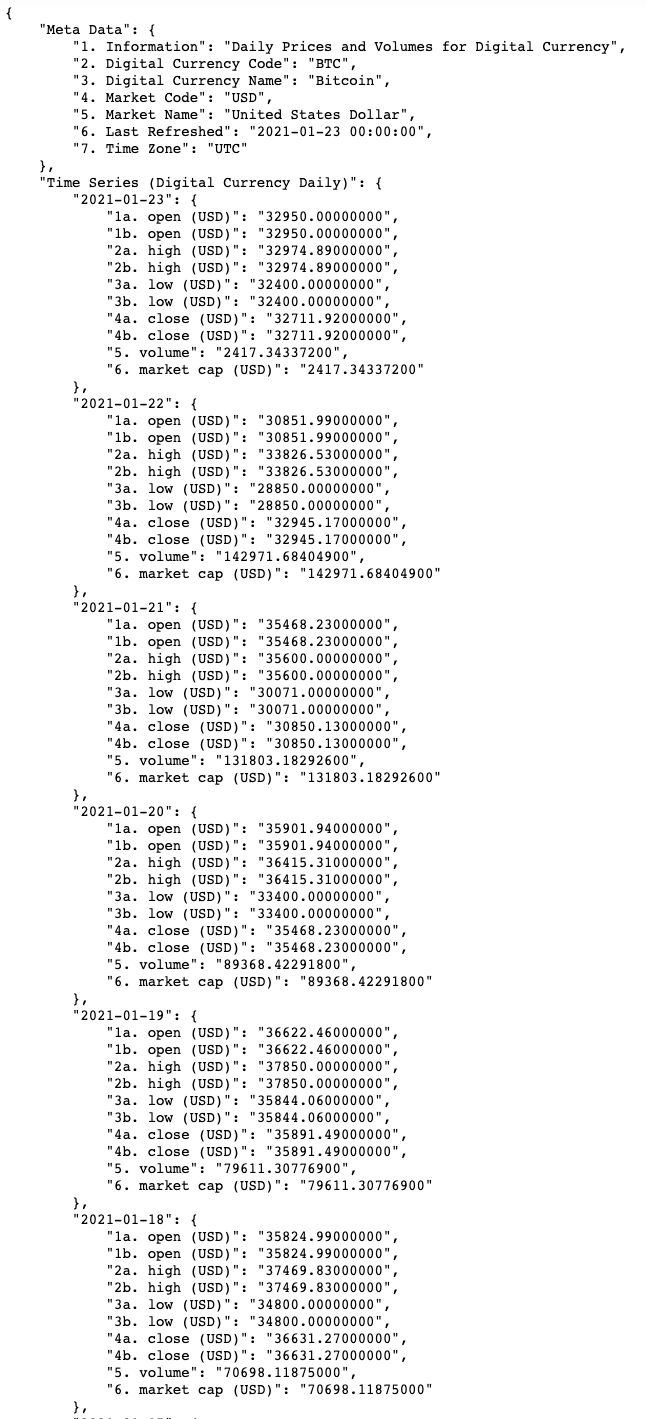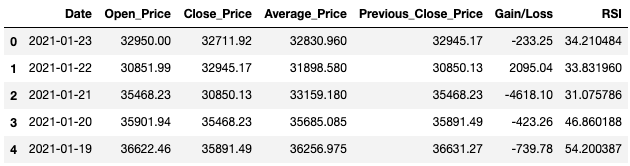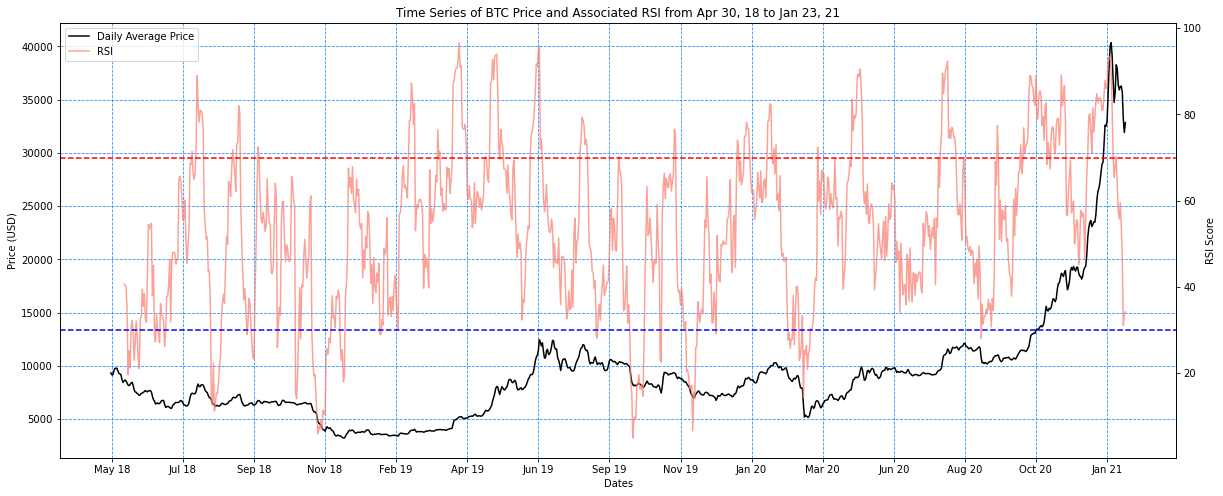Relative Strength Index Analysis on Time Series Data with Python
Jan 23, 21
Transforming and visualizing time series data to aid market trend predictions.Time series data, in the realm of finance, is useful for understanding past market behavior and predicting future trends. In this article, I will be demonstrating how to calculate and visualize the Relative Strength Index (RSI) from a time series that tracks an asset's price.

Relative Strength Index

The RSI is a technical analysis tool that can be used to indicate if an asset is overbought or oversold. A score between 0 to 100 is assigned to each data point in the time series, which is calculated as such:It is basically computing the relative strength as the average gains divided by the average loss over the lookback period and plugging it into the defined RSI equation. The first published formula used a lookback period of 14 days and so I will be sticking with that in my own implementation.

The traditional interpretation of RSI is that a score above 70 indicates that an asset is overbought (and possibly overvalued), and a score below 30 indicates that an asset is oversold (and possibly undervalued).

As a disclaimer, I do not recommend making buy or sell decisions based on RSI alone. RSI, and any other technical analysis tool, should be applied with great caution and understanding of the context in which it is used.

Python Libraries Used

``````import json
import requests
import pandas as pd
import matplotlib.pyplot as plt
import matplotlib.dates as mdates
from datetime import datetime
from statistics import mean``````

Obtaining the Time Series Data

I will be requesting daily price data from Alpha Vantage's free API. Specifically, I will be analyzing Bitcoin's price over time in USD. More details on using the API to obtain public financial data can be found in my previous article.

I have modified my function to retrieve data to include an additional argument called "market" as that is a required parameter to obtain digital currency data from the API.

``````def retrieve_data(function: str, symbol: str, api_key: str, market: str=None) -> dict:
"""
Retrieves data from AlphaVantage's open API.
Documentation located at: https://www.alphavantage.co/documentation
"""
# query from API
if market is None:
url = f'https://www.alphavantage.co/query?function={function}&symbol={symbol}&apikey={api_key}'
else:
url = f'https://www.alphavantage.co/query?function={function}&symbol={symbol}&market={market}&apikey={api_key}'
response = requests.get(url)
data = response.text
# parse output

return parsed``````

I then call my function with the required arguments to get Bitcoin's prices in the USD market. The full list of digital currencies and markets can be viewed from the respective links.

``````digital_currency = 'BTC'
market = 'USD'

currency_json = retrieve_data('DIGITAL_CURRENCY_DAILY', digital_currency, api_key, market)``````

Printing out the result of my request allows me to identify the specific data that is of interest to me. Here, I can see that I can use the given daily open and close prices for my analysis.I then extracted the relevant data and loaded it into a pandas Data Frame.

``````# extract relevant data
dates = []
open_price = []
close_price = []
for date, data in currency_json['Time Series (Digital Currency Daily)'].items():
dates.append(datetime.strptime(date, '%Y-%m-%d'))
open_price.append(mean([float(data['1a. open (USD)']), float(data['1b. open (USD)'])]))
close_price.append(mean([float(data['4a. close (USD)']), float(data['4b. close (USD)'])]))

# load relevant data into data frame
df_ts = pd.DataFrame({'Date': dates, 'Open_Price': open_price, 'Close_Price': close_price})``````

There needs to be a single price for each data point for me to chart a line graph of this data later on. I decided to take the average of the open and close price for each date as that single price point. Since the only numerical fields in my data frame are the open and close prices, I could simply apply the mean function across each row.

``df_ts['Average_Price'] = df_ts.mean(axis=1)``

Calculating the RSI

Before I could apply the RSI formula, I had to first calculate the gain or loss of each date from the previous date. I made sure my data was sorted from the latest date to the earliest date, then added the closing price shifted up by one row as a new column. This new column represents the closing price of the previous date. I then subtracted the closing price from the previous date from the reference date's closing price to derive the gain or loss.

``````# calculate gains/losses from previous day
df_ts['Previous_Close_Price'] = df_ts['Close_Price'].shift(periods=-1)
df_ts['Gain/Loss'] = df_ts['Close_Price'] - df_ts['Previous_Close_Price']``````

I could then iterate through each row in the data frame to calculate the respective RSI with a for loop. The DataFrame.iterrows() functionality in pandas produces the row index and the row data for each iteration.

``````# compute RSI
lookback_period = 14

rsi_list = []
for i, row in df_ts.iterrows():
try:
sum_gain = sum([df_ts.iloc[i + j]['Gain/Loss'] for j in range(lookback_period) if df_ts.iloc[i + j]['Gain/Loss'] > 0])
sum_loss = sum([-df_ts.iloc[i + j]['Gain/Loss'] for j in range(lookback_period) if df_ts.iloc[i + j]['Gain/Loss'] < 0])
average_gain = sum_gain/lookback_period
average_loss = sum_loss/lookback_period
relative_strength = average_gain/average_loss
rsi = 100 - (100/(1 + relative_strength))
except IndexError:
rsi = None
rsi_list.append(rsi)

df_ts['RSI'] = pd.Series(rsi_list)``````

Within each row, I calculated the average gains and losses over the prior 14 days (inclusive of the date of that row) and computed the relative strength. The relative strength was then plugged into the equation to obtain the RSI.

Notice how this calculation is wrapped in a try-except statement. The error being caught is an index out of bounds error. As there will not be a full 14 prior days of data for the earliest 13 dates in the data frame, the algorithm will be going outside of the data frame's index range to perform the RSI calculation for those dates. This error has to be caught and handled accordingly.

The data frame with the new column for RSI scores can be viewed once the loop has been completed.Visualization

Now that I have the price and the corresponding RSI scores for each date, I am ready to chart the data.

``````min_date = min(df_ts['Date']).strftime('%b %d, %y')
max_date = max(df_ts['Date']).strftime('%b %d, %y')

plt.figure(figsize=(20,8))
plt.title(f'Time Series of {digital_currency} Price and Associated RSI from {min_date} to {max_date}')
plt.xlabel('Dates')
plt.grid(color='dodgerblue', linestyle='--')

# plot price
ax = plt.gca()
ax.plot(df_ts['Date'], df_ts['Average_Price'], color='black', label='Daily Average Price')
ax.set_ylabel(f'Price ({market})')
ax.xaxis.set_major_formatter(mdates.DateFormatter('%b %y'))
ax.xaxis.set_major_locator(mdates.WeekdayLocator(interval=10))

# plot RCI
ax2 = ax.twinx()
ax2.plot(df_ts['Date'], df_ts['RSI'], color='salmon', alpha=70, label='RSI')
ax2.set_ylabel('RSI Score')
ax2.axhline(y=70, color='r', linestyle='--')
ax2.axhline(y=30, color='blue', linestyle='--')
ax2.xaxis.set_major_formatter(mdates.DateFormatter('%b %y'))
ax2.xaxis.set_major_locator(mdates.WeekdayLocator(interval=10))

# create legend
handles, labels = ax.get_legend_handles_labels()
handles2, labels2 = ax2.get_legend_handles_labels()
handles.extend(handles2)
labels.extend(labels2)
plt.legend(handles=handles, labels=labels, loc='upper left')

plt.show()``````

I plotted two axes - one of price vs date and one of RSI vs date - and overlay the second over the first to obtain a single chart. I also added two horizontal lines to demark the RSI scores of 30 and 70 to easily eyeball when Bitcoin's prices are likely underbought and overbought respectively.At a glance, it looks like the recent downtrend in Bitcoin is suggesting that the market could be running out of sellers.